KOA1.1
 Name:    KOA1.1

Multiple Choice
Identify the choice that best completes the statement or answers the question.

1.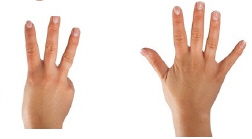How much do these fingers add uo to?
 a. 2 c. 9 b. 4 d. 8

2.

Which problem below is shown in this picture?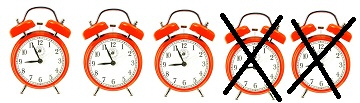a. 5 + 2 = 7 c. 2 + 3 = 5 b. 5 - 2 = 3 d. 7 - 2 = 5

3.

What is the answer to this equation?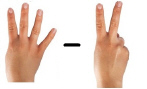a. 2 c. 6 b. 7 d. 5

4.

Which picture below shows this equation?  5 + 4
 a.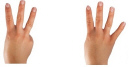c.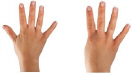b. 7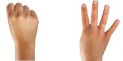d.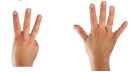5.

Which equation below would have the answer of 6?
 a. 6 + 2 c. 8 + 2 b. 9 - 3 d. 7 - 2